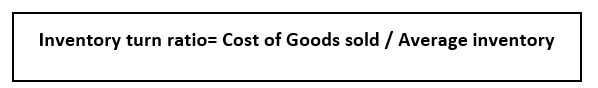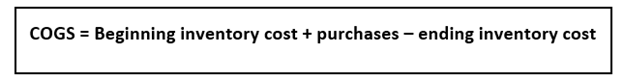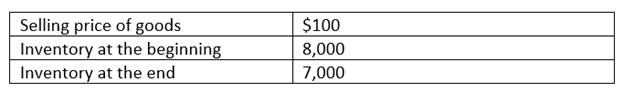# Inventory Turnover Ratio

• Updated on

## What is the inventory turnover ratio?

Inventory turnover ratio is an accounting ratio that establishes a relationship between revenue cost and the average inventory carried during an accounting period. It is an accounting ratio and is also commonly known as a stock turnover ratio.

The inventory turnover ratio is an important ratio for businesses as it explains how much stock carried by them is converted into sales. Simply put, the ratio describes the number of times the company sells its inventory during a given period.

The ratio is also becoming increasingly popular due to its importance in the ecommerce sector. Additionally, retail firms also use the ratio to keep a tab on their businesses. Turnover management is an essential step towards warehouse management.

## How is the ratio calculated?

The formula for inventory turns ratio is depicted below:In the above formula, the first step for the calculation is finding a time frame to measure the inventory turnover ratio. Now, one must calculate the cost of goods sold. This requires the stock count of the inventory at the beginning and end of the month. The following formula can be used to find the COGS:COGS can also represent revenue from operations, which refers to sales. Thus, sales can also be used in place of COGS to calculate inventory turnover ratio. Therefore, the cost of sales is the actual value of inventory converted to sales.

The second part of the formula involves finding out average inventory, which can be calculated using a simple formula. This involves adding the inventory stock at the beginning and end of the respective cycle then dividing it by 2.

The number obtained from the formula of inventory turnover ratio depicts the number of times the inventory is turned over within the time frame for which inventory change was measured.

## What is an example of inventory turnover ratio?

Consider a firm that wants to calculate inventory turnover ratio for the period of one month. The firm has the following information:Total cost of goods at the starting of the month is 100 * 8,000, which is equal to \$800,000. This is known as beginning inventory of the month. It is a good practice for companies to take inventory count by the end of the month again.

Thus, the total cost of goods at the end of the month is 100* 7000, equal to \$700,000. This is known as the ending inventory of the month.

Now the invoices suggest that a total of 7700 units of the good were purchased during this period of one month at the rate of \$100 per unit. This amounts to a total of \$770,000.

COGS can be calculated as: beginning inventory cost (800,000) + purchases (770,000) – ending inventory cost (700,000), this is equal to \$870,000. Therefore, COGS = \$870,000.

Average inventory cost can be calculated simply by taking the average of beginning and ending inventory. Thus, average inventory cost = (800,000+ 700,000)/2 = \$750,000.

Finally, total inventory turnover can be calculated by dividing COGS by average inventory cost. This gives the value 870,000/750,000 = 1.16.

This means that the company turns in the inventory once every month.

## What does the ratio signify?

The inventory turns ratio signifies how many times the inventory is turned in within a specific period, which was one month in the above case. This ratio can be compared with competitor firms to understand better how a company is performing.

Suppose that a competitor of the firm has an inventory turns ratio of 2.5, then clearly the other firm is selling more products than the concerned firm. However, it is important to note that this ratio alone cannot deem a business as inefficient or worse than its competitors. Other metrics may turn out to be higher for a firm with a lower inventory turns ratio.

Additionally, inventory turns ratio can be used to calculate how many days its takes for a firm to finish its inventory. This can simply be achieved by dividing 365 by the inventory turnover ratio. In the above case it would take 314 days for the firm to completely clear its inventory.

## Why is the inventory turnover ratio important?

A high inventory turnover ratio signifies how successful a company is in turning stock into sales. It also suggests that the company is actively partaking in inventory control measures, coupled with a strong sales policy.

Low turnover ratio points to lack of demand for the company’s goods. It may also hint at outdated products being sold by the company. This could mean a piling up of stocks in the future for the company. Moreover, the quality of a good may deteriorate over time as it is kept it storage.

An ideal inventory ratio for a company can vary depending on the size and type of company it is. However, it is a good policy for a company to clean out its inventory 12-13 times a year.

## What are the limitations of this ratio?

Despite its many advantages, the inventory turns ratio comes with certain limitations too. The time taken by a company to sell its inventory can vary greatly depending on the type of product that is sold. Thus, having a fair idea about the inventory turns for the respective industry can give a better idea about how well the business is doing.

Consider the case of fast-moving goods wherein its easier for firms to make sales as goods are lower priced and come with a shelf life. Consequently, if a retail chain selling everyday supplies has a high inventory turns ratio, then it does not necessarily mean that the firm is performing exceedingly well. It is just the nature of the industry that the firm is a part of.

On the contrary, consider the case of real estate sector, wherein a property may take time to get sold. However, from the sale of a single property, high amount of revenue can be generated.

##### Top ASX Listed Companies

Definition:
We use cookies to ensure that we give you the best experience on our website. If you continue to use this site we will assume that you are happy with it. OK